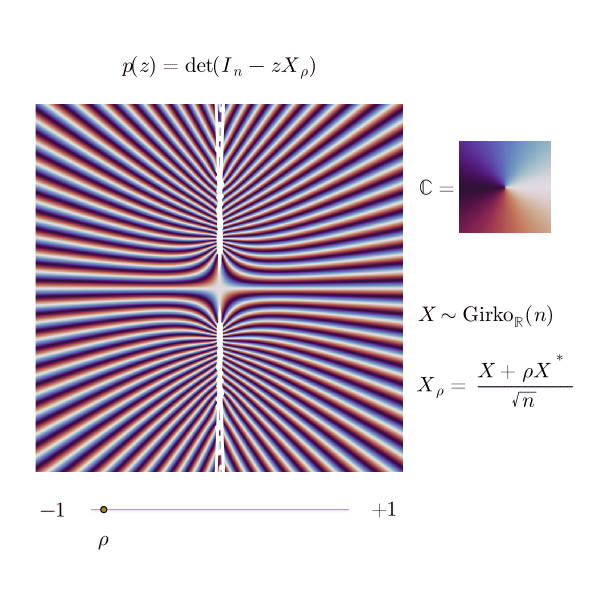# An inverse visualization for the elliptic law

March 2021

Here are two nice facts on spectra of random matrices of large size $n$.

• When $A$ is a Girko matrix, the eigenvalues of $A/\sqrt{n}$ asymptotically follow the circular law – they tend to be uniformly distributed in $\mathbb{D} = D(0,1)$. By Girko matrix, I mean a matrix where all the entries are iid real random variables, centered, with variance 1.

• When $A$ is a Wigner matrix, the eigenvalues of $A/\sqrt{n}$ asymptotically follow a semi-circle law, and in particular they are real. By Wigner matrix, I mean a symmetric matrix where all the entries on and above the diagonal are iid real random variables, centered, with variance 1.

What happens in between lies in the elliptic realm. If $A$ is a Wigner matrix, the symmetry of $A$ is indeed equivalent to $A$ being maximally correlated with its own transpose $A^*$, since they are equal. One can thus create intermediary models between Girko and Wigner, by parametrizing the degree of correlation above and below the diagonal: for this, we just take $A$ to be a fully independent matrix (Girko), and set

$X_\rho = A + \rho A^*.$

The matrix $X_0 = A$ is a Girko matrix; the matrix $X_1 = A + A^*$ is a symmetric matrix and the matrix $X_{-1} = A - A^*$ it is an antisymmetric matrix. In $X_\rho$, the entries above and below the diagonal are correlated, in that if $i \neq j$,

$\mathrm{Cov}((X_\rho)_{i,j},(X_\rho)_{i,j}) = 2\rho\mathrm{Var}(A_{i,j}) = 2\rho.$

It turns out that the eigenvalues of $X_\rho$ asymptotically follow the elliptic distribution: they tend to be uniformly distributed inside an ellipse, ie the domain defined by

$\frac{\mathrm{Real}(z)^2}{(1 + \rho)^2} + \frac{\mathrm{Imag}(z)^2}{(1 - \rho)^2} \leqslant 1.$

The animated picture below is an illustration of this phenomenon, as seen from $\infty$.For several correlation parameters, I represented the phase portrait of the reciprocical of the characteristic determinant,

$q_n(z)=\det(I - zX_\rho / \sqrt{n})$

as a complex function; the white dots are the inverses of the eigenvalues of $X_\rho/\sqrt{n}$, and the white line is the inverse of the ellipse.

## Polynomial convergence

The complex polynomial $q_n$ has degree $n$. Its (complex) roots are the inverses of the (complex) eigenvalues of $A/\sqrt{n}$. The central picture in the preceding animation, with $\rho=0$, illustrates a convergence phenomenon regarding $q_n$, which recently appeared in a beautiful paper by Bordenave, Chafaï and García-Zelada.

They showed that if $A$ is a real Girko matrix entries centered and reduced, then

$q_n \xrightarrow[n \to \infty]{\mathrm{law}} \kappa \mathrm{e}^{-F}$

where $\kappa, F$ are holomorphic functions; $\kappa$ is deterministic,

$\kappa(z) = \sqrt{1 - z^2},$

while $F$ is itself a random function,

$F(z) = \sum_{\ell = 1}^\infty X_\ell \frac{z^\ell}{\sqrt{\ell}}$

where the $X_\ell$ are iid standard real Gaussian random variables. The mode of convergence in (5) is the weak convergence of probability measures on the space $\mathbb{H}(\mathbb{D})$ – the space of holomorphic functions on the open unit disk, endowed with the classical topology of uniform convergence on compact sets.

Since the limiting random function $z \mapsto \kappa(z)\mathrm{e}^{-F(z)}$ does not vanish inside $\mathbb{D}$, one can use results like the Hurwitz theorem to show that when $n$ is large, it is highly unlikely that $q_n$ has a root inside $D(0, 1-\epsilon)$ – thus proving that the eigenvalue of $A/\sqrt{n}$ with highest modulus, say $\lambda_1$, has $\limsup |\lambda_1| \leqslant 1 + \epsilon$ with probability $1-o(1)$.

This is already visible in the picture corresponding to $\rho=0$ above, even though $n=100$ is pretty small here; the inverse eigenvalues seem to avoid the disk $\mathbb{D}$, or be very close to its boundary. Looking at the other pictures, one would merrily suppose that a similar statement holds for every $\rho$ between $-1$ and $1$, with no eigenvalue of $X_\rho/\sqrt{n}$ being really far away outside of the ellipse boundary.

## Notes and References

The original paper of Girko on the elliptic law... in Russian.

Convergence of the spectral radius of a random matrix through its characteristic polynomial, Bordenave, Chafaï, García-Zelada.

Comments on the circular law, a blogpost by Djalil Chafaï on open problems and recent works around the circular law.

My Julia code for the animated picture.

  I will stick to random matrices having real entries, but a similar picture holds with complex entries.